13 January 20222329
Reasuransi Umum

## Supervised and Unsupervised, an Introduction to Machine Learning

Machine Learning is a subset of Artificial Intelligence technique that uses statistical method to enable machines to improve or expand it self with experience.In the 90s, Arthur Samuel defined machine learning as follows: "It is a field of study that gives the ability to the computer for self-learn without being explicitly programmed". This means that machine learning is able to provide knowledge to itself, to learn independently without a special programming language

Why we need Machine Learning…???. The role of machine learning really supports the development of the current digitalization era, assists the decision-making process, provides information through highly interactive visualizations and able to predict events by comparing accuracy values. Machine learning can also execute job automation, perform big data-based model analysis with good accuracy. Figure 1 shows the illustration of Machine Learning Workflow.Figure. 1. Machine Learning Workflow

There are two kinds of machine learning algorithms that are very commonly used, namely Supervised and Unsupervised Learning.
1. Supervised Learning

The main characteristic of supervised learning is the "label/outcome (y)" that must be provided in our data, machine will learn to generate a new data pattern and then make predictions by given a certain error value as a measurement of selecting the best model. One of the basic types of supervised learning is Regression. Regression is the process of estimating the relationship between input (x) and output (y) variables. Regression analysis helps us in understanding how the value of the output variable changes when we vary some input variables while keeping other input variables fixed ().

We have usually assumed Regression Model (Linear) as below :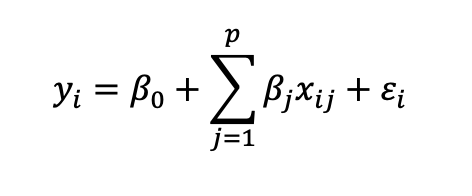Another supervised learning method is Classification. Classification is a method to analyze the relationship between several predictor variables and one response variable / label. Classification solves the problem of identifying the category to which a new data point belong. This is used extensively in spam identification, face recognition, recommendation engines, and so on. The algorithms for data classification will come up with the right criteria to separate the given data into the given number of classes.

Figure 2 shows a graphical summary of Supervised Learning.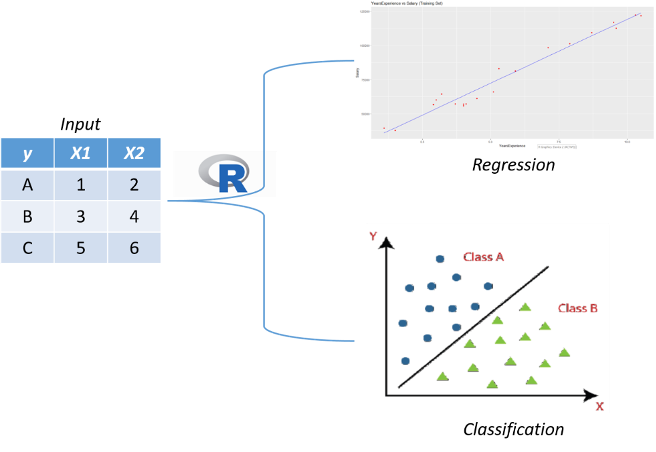Figure. 2. Supervised Learning

1. Unsupervised Learning

The next type is Unsupervised Learning, in contrast to supervised learning, this method does not require a label/outcome (y). The most commonly used unsupervised learning in machine learning is Cluster analysis. Clustering is used to perform 'grouping' based on the same characteristic information, another benefit are very useful to reduce the number of problem sizes and complexity for data mining methods (dimension reduction) (). See for an illustration of Unsupervised Learning using Cluster Analysis.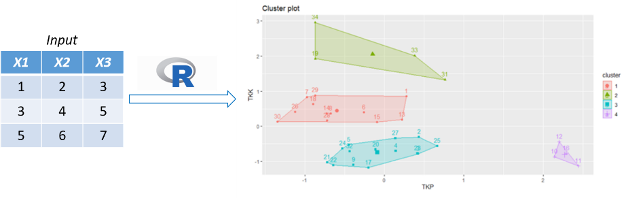Figure. 3. Unsupervised Learning

The purpose of cluster analysis is to group similar objects together. Objects that have a smaller distance will be considered the same or similar in one group compared to other objects with a greater distance (Gower et al., 2010).
There are some commonly used distances:

1. Euclidean distance :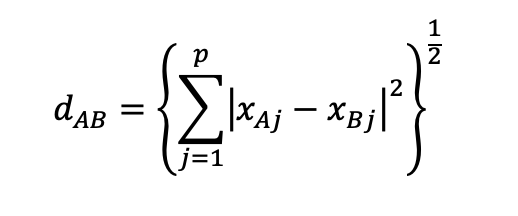p = many measurement variables

1. Manhattan distance :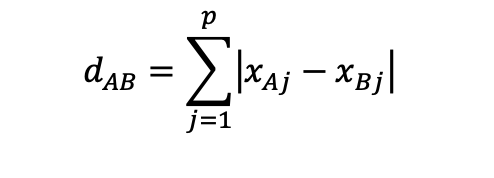1. Spearman distance :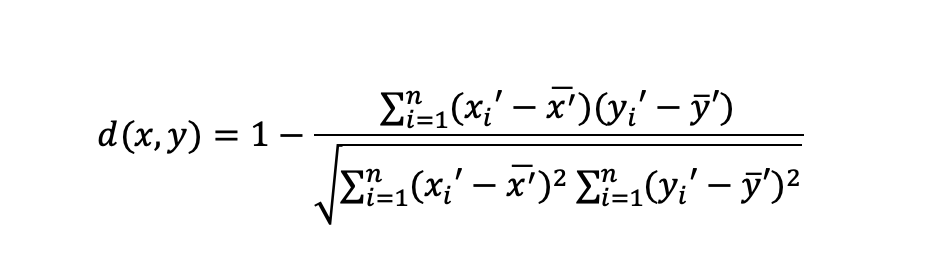From the explanation above, hopefully it can give value added to our insight about criteria for machine learning methods. See you in the next chapter.

Reference:
Jamilatuzzahro, Caraka R, Riki H. 2018. Aplikasi Generalized Linear Model pada R. Yogyakarta: Innosain
Caraka RE, Lee Y, Chen RC, et al (2021) Cluster around Latent Variable for Vulnerability towards Natural Hazards, Non-Natural Hazards, Social Hazards in West Papua. IEEE Access 9:1972–1986. https://doi.org/10.1109/ACCESS.2020.3038883
Gower J, Lubbe S, Roux N le (2010) Biplot Basics. In: Understanding Biplots. pp 11–66

## Penulis### Faisal Anggoro, S.Si., M.E.

Email: faisal@indonesiare.co.id